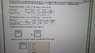# Rate Law - Isolation Method

cvc121

## Homework Statement

Due to formatting problems, I have attached the problem below.

## The Attempt at a Solution

Plotting the appropriate graphs and based on linearity, I have determined that the reaction is first order with respect to [O2] and second order with respect to [NO]. Therefore, the reaction is third order overall and this corresponds to M-2s-1 as the units for the rate constant, k.

However, I am unsure of how to solve for the rate constant. I know that this is an isolation method problem where the initial concentration of one reactant is much smaller than the initial concentration of the others. As such, the large concentrations barely change during the reaction and are approximately constant.

I also know that I will be needing the slopes of the graphs. For the graph of [O2] in experiment 1, the slope is 0.2328. The slope of the graph of [NO] for the second experiment is 0.0000238.

For instance, in experiment 1, the concentration of NO would stay approximately constant throughout the reaction because it is so large relative to the concentration of [O2]. So I could write the rate constant as R = kobs (observed rate constant - corresponds to slope of the graph) [O2].

Do I just plug in the numbers? Using experiment 1:
k = observed rate constant / [O2] = 0.2328 / 4.10 x 10-4 = 567.8 M-2s-1?

I do not think this is correct because going through the same process for experiment 2, I get a different result.

Can anyone provide some guidance? Thanks. All help is very much appreciated.

#### Attachments

•20170308_103852.jpg
47.6 KB · Views: 617

Homework Helper
Compare your equation R = kobs[O2] with the true rate equation. What is kobs equal to?

cvc121
Compare your equation R = kobs[O2] with the true rate equation. What is kobs equal to?

My true rate equation is R = k [NO]2[O2]. Therefore, the observed rate constant, kobs, is equal to k [NO]2. Rearranging this, I get k = kobs / [NO]2. So do I plug the values from experiment 1 into this equation to get the actual rate constant?

k = 0.2328 / [9.63 x 10-1]2 = 0.251 M-2s-1?